# Bernoulli Naive Bayes

## Introduction

To understand Bernoulli Naive Bayes algorithm, it is essential to understand Naive Bayes.

Naive Bayes is a supervised machine learning algorithm to predict the probability of different classes based on numerous attributes. It indicates the likelihood of occurrence of an event. Naive Bayes is also known as conditional probability.

Naive Bayes is based on the Bayes Theorem.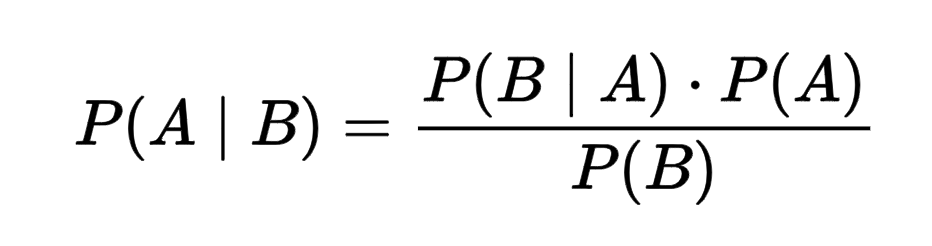where:-

A: event 1

B: event 2

P(A|B): Probability of A being true given B is true - posterior probability

P(B|A): Probability of B being true given A is true - the likelihood

P(A): Probability of A being true - prior

P(B): Probability of B being true - marginalization

However, in the case of the Naive Bayes classifier, we are concerned only with the maximum posterior probability, so we ignore the denominator, i.e., the marginal probability. Argmax does not depend on the normalization term.

The Naive Bayes classifier is based on two essential assumptions:-

(i) Conditional Independence - All features are independent of each other. This implies that one feature does not affect the performance of the other. This is the sole reason behind the ‘Naive’ in ‘Naive Bayes.’

(ii) Feature Importance - All features are equally important. It is essential to know all the features to make good predictions and get the most accurate results.

Naive Bayes is classified into three main types: Multinomial Naive Bayes, Bernoulli Naive Bayes, and Gaussian Bayes.

We will be talking about Bernoulli Naive Bayes in this blog.

Before going ahead, let us have a look at the Bernoulli Distribution:-

Let there be a random variable 'X' and let the probability of success be denoted by 'p' and the likelihood of failure be represented by 'q.'

Success: p

Failure: q

q = 1 - (probability of Sucesss)

q = 1 - p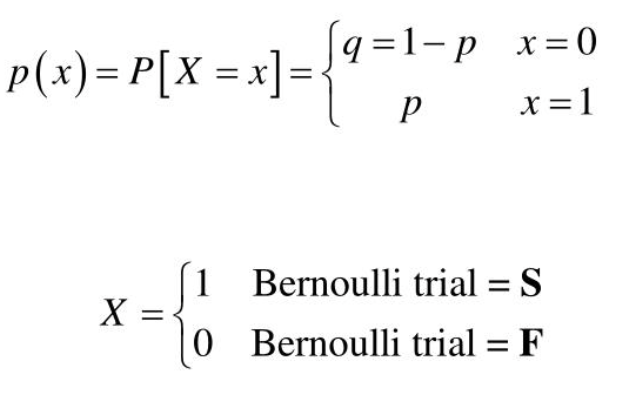As we notice above, x can take only two values (binary values), i.e., 0 or 1.

Bernoulli Naive Bayes is a part of the Naive Bayes family. It is based on the Bernoulli Distribution and accepts only binary values, i.e., 0 or 1. If the features of the dataset are binary, then we can assume that Bernoulli Naive Bayes is the algorithm to be used.

Example:

(i) Bernoulli Naive Bayes classifier can be used to detect whether a person has a disease or not based on the data given. This would be a binary classification problem so that Bernoulli Naive Bayes would work well in this case.

(ii) Bernoulli Naive Bayes classifier can also be used in text classification to determine whether an SMS is ‘spam’ or ‘not spam.’

## Mathematics Behind

Let us consider the example below to understand Bernoulli  Naive Bayes:-

In the above dataset, we are trying to predict whether a person has a disease or not based on their age, gender, and fever. Here, ‘Disease’ is the target, and the rest are the features.

All values are binary.

We wish to classify an instance ‘X’ where Adult=’Yes’, Gender= ‘Male’, and Fever=’Yes’.

Firstly, we calculate the class probability, probability of disease or not.

P(Disease = True) = ⅗

P(Disease = False) = ⅖

Secondly, we calculate the individual probabilities for each feature.

P(Adult= Yes | Disease = True) = ⅔

P(Gender= Male | Disease = True) = ⅔

P(Fever= Yes | Disease = True) = ⅔

P(Adult= Yes | Disease = False) = ½

P(Gender= Male | Disease = False) = ½

P(Fever = Yes | Disease = False) = ½

Now, we need to find out two probabilities:-

(i) P(Disease= True | X) = (P(X | Disease= True) * P(Disease=True))/ P(X)

(ii) P( Disease = False | X) = (P(X | Disease = False) * P(Disease= False) )/P(X)

P(Disease = True | X) = (( ⅔ *⅔ * ⅔ ) * (⅗))/P(X) = (8/27 * ⅗) / P(X)  = 0.17/P(X)

P(Disease = False | X) = [(½ * ½ * ½ ) * (⅖)] / P(X)  = [⅛ * ⅖] / P(X)  = 0.05/ P(X)

Now, we calculate estimator probability:-

P(X) = P(Adult= Yes) * P(Gender = Male ) * P(Fever = Yes)

= ⅗ * ⅗ * ⅗ = 27/125 = 0.21

So we get finally:-

P(Disease = True | X) = 0.17 / P(X)

= 0.17 / 0.21

= 0.80 - (1)

P(Disease = False | X) = 0.05 / P(X)

= 0.05 / 0.21

= 0.23  - (2)

Now, we notice that (1) > (2), the result of instance ‘X’ is ‘True’, i.e., the person has the disease.

## Dataset

The dataset we're using will be helpful in SMS Message Spam Detection. It consists of 4 features and one target variable.

(i) message - text message, categorical feature.

(ii) Unnamed:2 - unknown feature, we will be dropping this.

(iii) Unnamed:3 - unknown feature, we will be dropping this.

(iv) Unnamed:4 - unknown feature, we will be dropping this.

(v) class - target variable, binary feature - ‘spam’ or ‘ham’ .

## Implementation

For self-implementation, we will have to create three functions, one for estimating prior probability, one for estimating conditional probability, and one for prediction. The working of the same has been discussed in the previous section.

For simplicity, we would be using the already existing sklearn library for Bernoulli Naive Bayes implementation.

## Importing Necessary Libraries

Firstly, we will load some basic libraries:-

(i) Numpy - for linear algebra.

(ii) Pandas - for data analysis.

(iii) Seaborn - for data visualization.

(iv) Matplotlib - for data visualisation.

(v) BernoulliNB - for Bernoulli Naive Bayes implementation.

(vi) CountVectorizer - for sparse matrix representation.

## Visualization

We visualize the dataset by printing the first ten rows of the data frame. We use the head() function for the same.

Output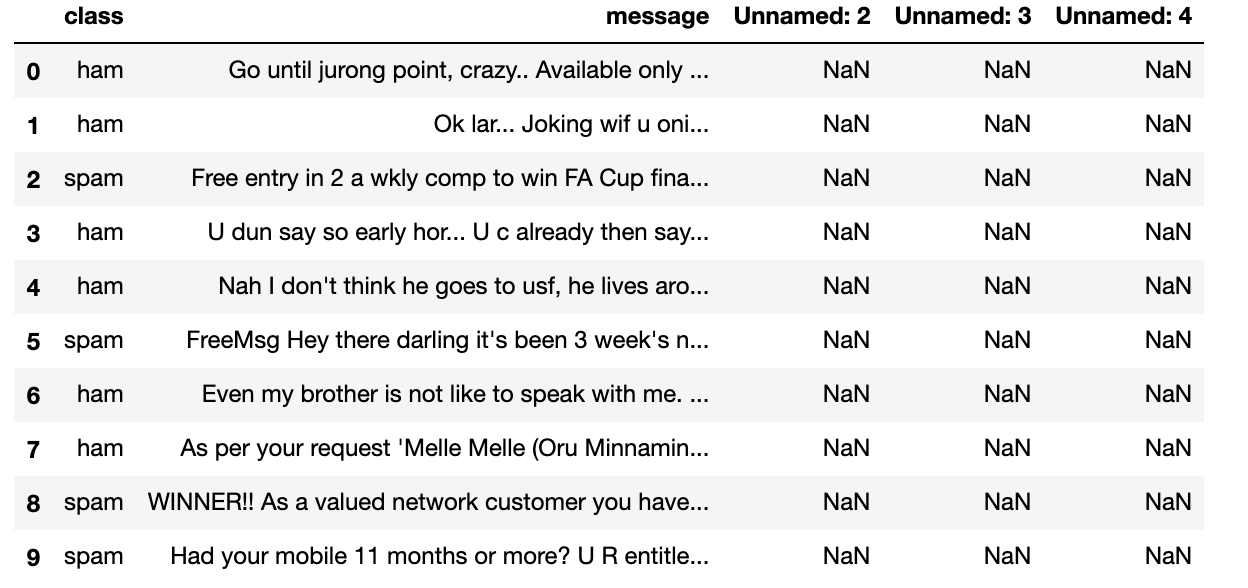Above, we observe all the features and the target variable 'class.' Also, we notice that three columns 'Unnamed:2', 'Unnamed:3' and 'Unnamed:4' contain many NaN or missing values. We will be handling the same in the next section.

Now, we use the shape function to get an idea about the dimensions of the dataset.

Output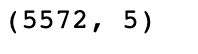From the above, we observe there are 5572 examples and five columns.

## Preprocessing

1. Data imputation

We drop 'Unnamed:2', 'Unnamed:3' and 'Unnamed:4' as they contain too many missing values. Also, these features are unknown, so there is no point in retaining them.

Output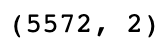We notice that three features have been dropped, and now our data contains just two columns, one representing the 'message' feature and one representing the 'class' target variable.

2. Binarization

To test Bernoulli Naive Bayes on the dataset, we need to ensure that all values are binary.

So, firstly, we check if our target variable values are binary or not.

Output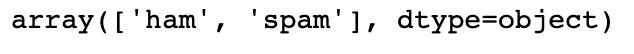We notice that our target variable has binary values, 'ham' or 'spam.'

Secondly, we check if our ‘message’ feature values are binary or not.

Output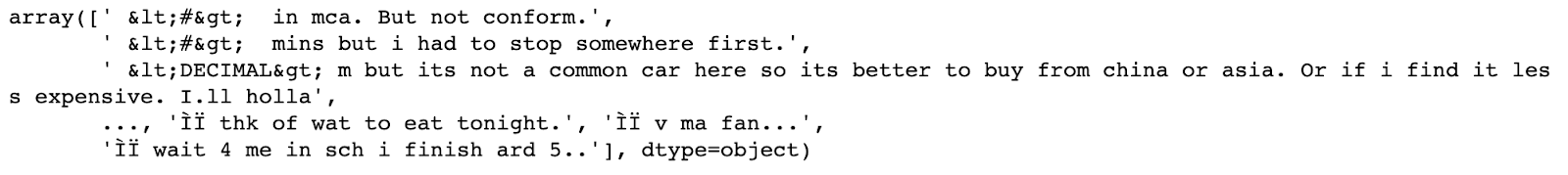We notice that our ‘message’ feature is not binary. So we will use CountVectorizer to fix this.

3. Vectorization

Now, we will use CountVectorizer() to fix our 'message' feature by creating a sparse matrix.

Output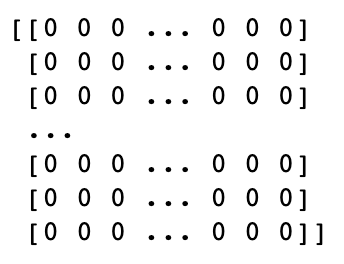4. Data arrangement

Now, we will just arrange our dataset such that our target variable is the last column. This will make training easier.

Output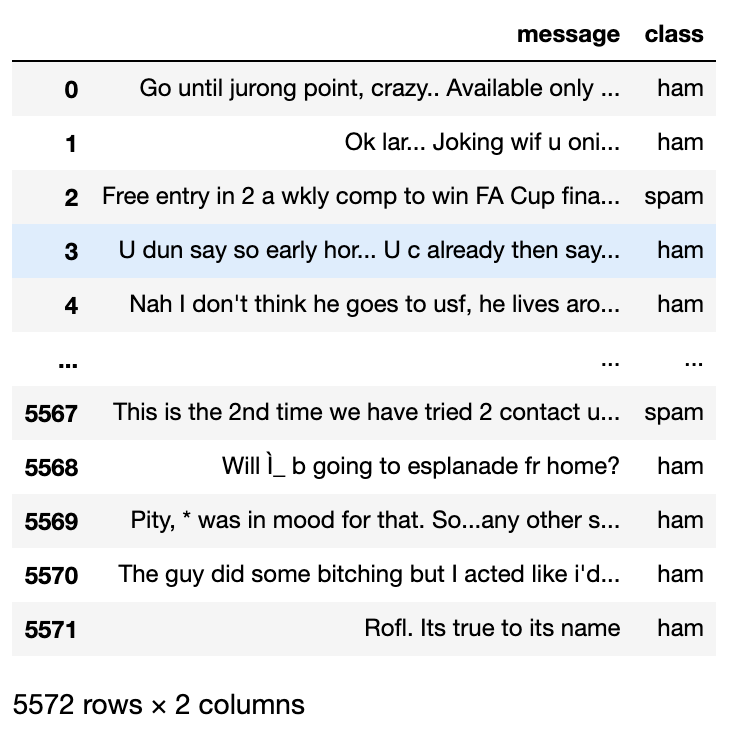5. Train-Test Split

Now, we will divide our data into training data and testing data. We will have a 3:1 train test split. This would imply that our training data will have 4179 examples, whereas our testing data will have 1393 examples.

### Training

We will build our Bernoulli Naive Bayes model using the sklearn library and then train it.

We have passed 'binarize' as a parameter for binarizing the values of the dataset. We have also generated the prediction, and now we will move on to the results.

## Results

Now, we analyze our model and generate the results.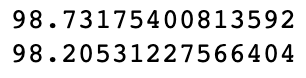We notice that we get good results on both training and testing sets. The training set gives us a score of 98.73, whereas the testing set gives us a score of 98.20.

Now, we will also generate classification reports for training and testing sets.

For training set:-

Output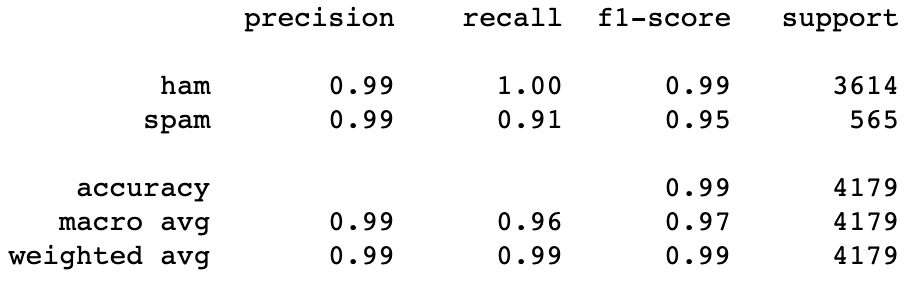For testing set:-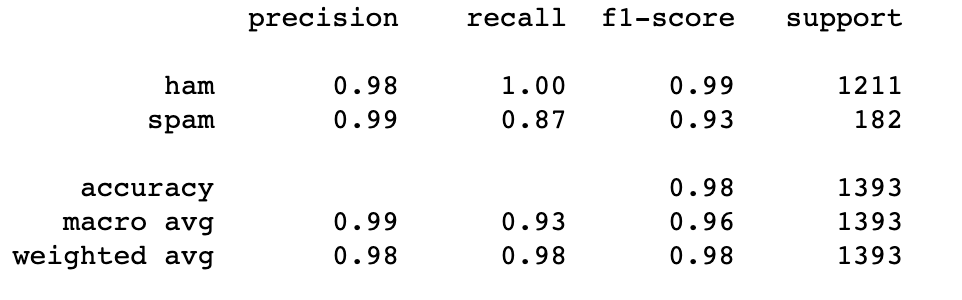As visible from the above, we have been able to get good results. Finally, we are done studying Bernoulli Naive Bayes.

1. How many types of Naive Bayes Classifiers are there?
Naive Bayes can be classified into three types:-
(i)  Multinomial Naive Bayes- suitable for discrete features.
(ii) Bernoulli Naive Bayes - suitable for binary features.
(iii) Gaussian Naive Bayes - suitable for continuous features.

2. What is the limitation of the Naive Bayes Classifier?
The main limitation of the Naive Bayes classifier is its assumption of conditional independence - all features are independent of each other. In reality, this is highly improbable.

3. What is the advantage of the Naive Bayes Classifier?
The main advantage of the Naive Bayes classifier is that it is really fast in the case of multi-class predictions. When it comes to classification, it performs better than other models such as Logistic Regression.

## Key Takeaways

Congratulations on making it this far. This blog discussed a fundamental overview of the Bernoulli Naive Bayes Classifier!!

We learned about Data Loading, Data Visualisation, Data Preprocessing, and Training. We learned how to visualize data then, based on this EDA, took significant decisions concerning preprocessing, made our model training ready, and finally generated the results.

If you are preparing for the upcoming Campus Placements, don’t worry. Coding Ninjas has your back. Visit this link for a carefully crafted and designed course on-campus placements and interview preparation.